# Visualizing Tabular Data

Lesson 2 with Elizabeth Green

## Lesson Objectives

• Meet the “grammar of graphics” for ggplot2
• Trust us: this is better than base R’s plot
• Learn to layer visual elements on top of tidy data
• Glimpse the vast collection of ggplot2 options

## Specific Achievements

• Create “aesthetic mappings” between variables and geometries
• Build boxplots, scatterplots, smoothed lines and histograms
• Style plots with colors, annotate them with labels
• Repeat plots for different subsets of data

Top of Section

## Getting Started

Let’s start by loading a few packages along with a sample dataset, which is the animals table from the Portal Project Teaching Database.

library(dplyr)
na.strings = '') %>%
select(year, species_id, sex, weight) %>%
na.omit()


Omitting rows that have missing values for the species_id, sex, and weight columns is not strictly necessary, but it will prevent ggplot from returning missing values warnings.

### Layered Graphics

As a first example, this code plots each invidual’s weight by species:

library(ggplot2)
ggplot(animals,
aes(x = species_id, y = weight)) +
geom_point()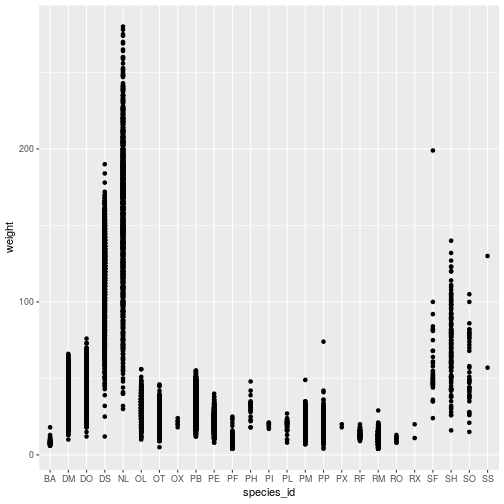The ggplot command expects a data frame and an aesthetic mapping. The aes function creates the aesthetic, a mapping between variables in the data frame and visual elements in the plot. Here, the aesthetic maps species_id to the x-axis and weight to the y-axis.

The ggplot function by itself does not display anything until we add a geom_* layer, in this example a geom_point. Layers are literally added, with +, to the object created by the ggplot function.

Individual points are hard to distinguish in this plot. Might a boxplot be a better visualization? The only change needed is in the geom_* layer.

ggplot(animals,
aes(x = species_id, y = weight)) +
geom_boxplot()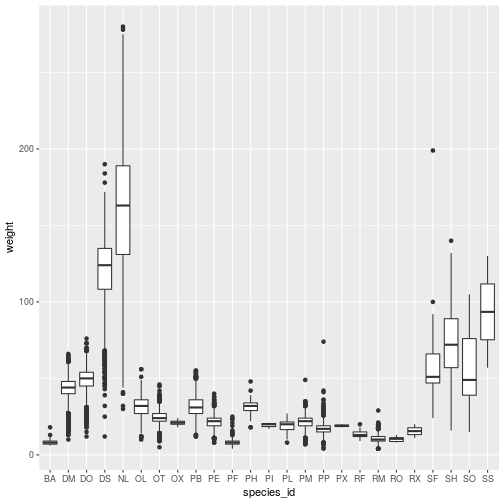Add geom_* layers together to create a multi-layered plot:

ggplot(animals,
aes(x = species_id, y = weight)) +
geom_boxplot() +
geom_point()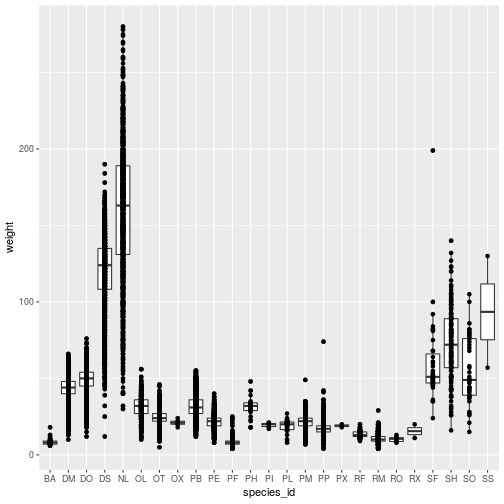Each geom_* object accepts arguments to customize that layer. Many arguments are common to multiple geom_* functions, such as those for adding blanket styling to the layer.

ggplot(animals,
aes(x = species_id, y = weight)) +
geom_boxplot() +
geom_point(color = 'red')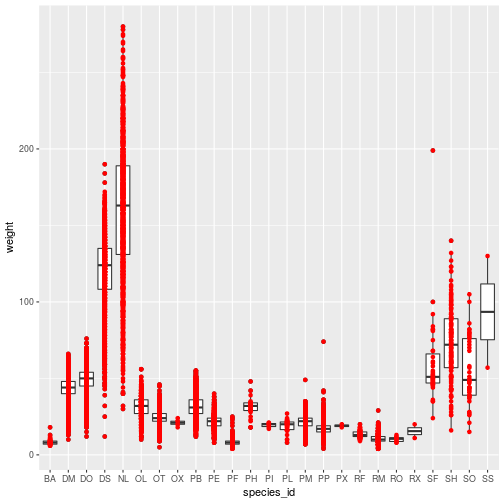The color specification was not part of aesthetic mapping between data and visual elements, so it applies to the entire layer.

The stat parameter, in conjunction with fun.y, provide the ability to perform on-the-fly data transformations.

ggplot(animals,
aes(x = species_id, y = weight)) +
geom_boxplot() +
geom_point(
color = 'red',
stat = 'summary',
fun.y = 'mean')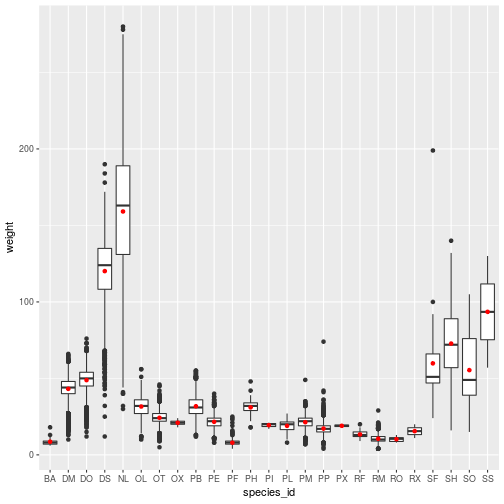The geom_point layer definition illustrates two features:

• With stat = 'summary', the plot replaces the raw data with the result of a summary function, defined by fun.y.
• Setting color = red applies one color to the whole layer.

Associating color (or any attribute, like the shape of points) to a variable is another kind of aesthetic mapping. Passing the color argument to the aes function works quite differently than assiging color to a geom_*.

ggplot(animals,
aes(x = species_id, y = weight,
color = species_id)) +
geom_boxplot() +
geom_point(stat = 'summary',
fun.y = 'mean')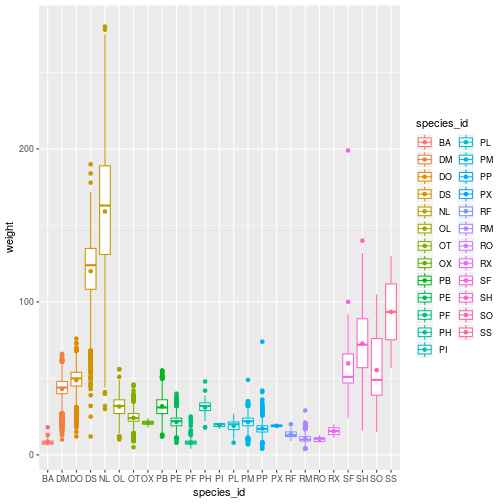Top of Section

## Smooth Lines

The geom_smooth layer adds a regression line with confidence intervals (95% CI by default). The method = 'lm' parameter specifies that a linear model is used for smoothing.

Load some data you might use for a linear model with a categorical predictor of a continuous response.

levels(animals\$sex) <- c('Female', 'Male')
animals_dm <- filter(animals,
species_id == 'DM')


With a categorical predictor mapped to an aesthetic element, the geom_smooth call will separately apply the lm method. The result hints at the significance of the predictor.

ggplot(animals_dm,
aes(x = year, y = weight, shape = sex)) +
geom_point(size = 3,
stat = 'summary', fun.y = 'mean') +
geom_smooth(method = 'lm')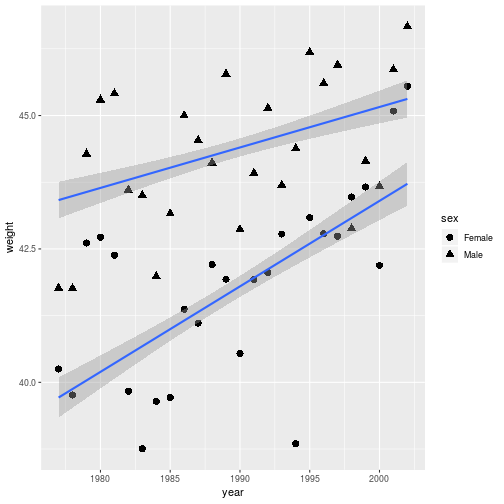Even better would be to distinguish everything (points and lines) by color.

ggplot(animals_dm,
aes(x = year, y = weight,
shape = sex, color = sex)) +
geom_point(size = 3,
stat = 'summary', fun.y = 'mean') +
geom_smooth(method = 'lm')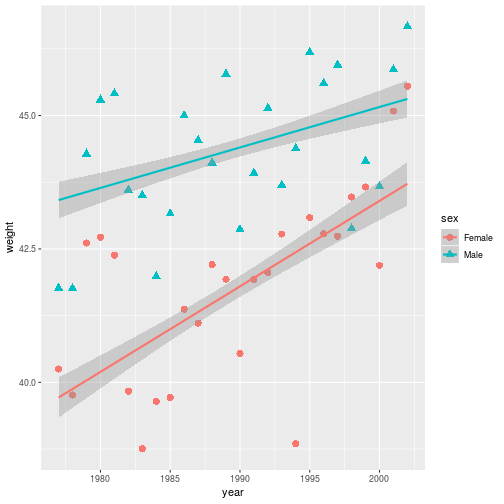Notice that by adding aesthetic mappings in the base aesthetic (in the ggplot command), it is applied to any layer that recognizes the parameter.

Top of Section

## Storing and Re-plotting

The output of ggplot can be assigned to a variable. It is then possible to add new elements to it with the + operator. We will use this method to try different color scales for a stored plot.

year_wgt <- ggplot(animals_dm,
aes(x = year, y = weight,
color = sex, shape = sex)) +
geom_point(size = 3,
stat = 'summary',
fun.y = 'mean') +
geom_smooth(method = 'lm')


The plot information stored in year_wgt can be used on its own, or with additional layers.

> year_wgtBy overwriting the year_wgt variable, the stored plot gets updated with the black and red color scale.

year_wgt <- year_wgt +
scale_color_manual(
values = c('black', 'red'))

> year_wgt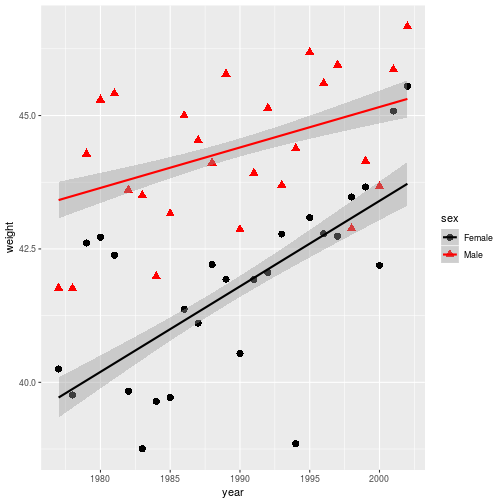Figures are constructed in ggplot2 as layers of shapes, from the axes on up through the geom_* elements. Natural file types for storing these figures at “infinite” resolution are PDF (for print) or SVG (for online).

ggsave(filename = 'year_wgt.pdf',
plot = year_wgt,
width = 4, height = 3)


The plot argument is unnecessary if the target is the most recently displayed plot. When a raster file type is necessary (e.g. a PNG, JPG, or TIFF) use the dpi argument to specify an image resolution.

Top of Section

## Axes, Labels and Themes

Let’s start looking at annotation and other customizations on a new geom_*, one that creates a histogram. Due to the nature of histograms, the base aesthetic does not require a mapping for y.

histo <- ggplot(animals_dm,
aes(x = weight, fill = sex)) +
geom_histogram(binwidth = 3,
color = 'white')

> histo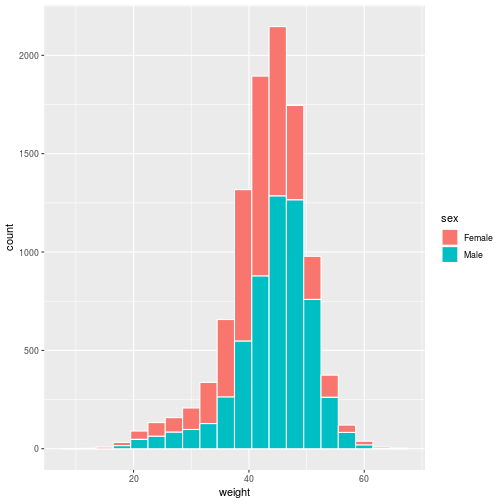Set the title and axis labels with the labs function, which accepts names for labeled elements in your plot (e.g. x, y, title) as arguments.

histo <- histo + labs(title =
'Size of Dipodomys merriami',
x = 'Weight (g)',
y = 'Count')

> histo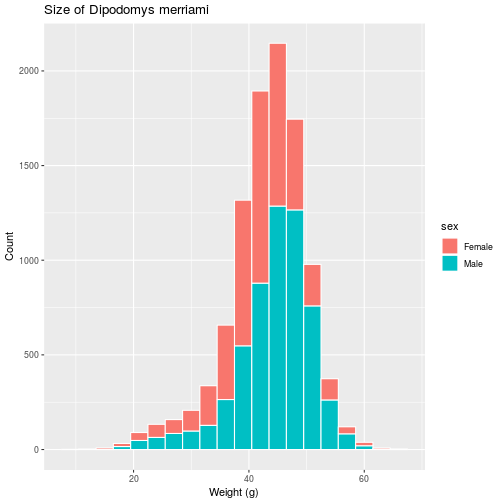For information on how to add special symbols and formatting to plot labels, see ?plotmath.

We have various functions related to the scale of each axis, i.e. the range, breaks and any transformations of the values on the axis. Here, we use scale_x_continuous to modify the continuous (as opposed to discrete) x-axis.

histo <- histo + scale_x_continuous(
limits = c(20, 60),
breaks = c(20, 30, 40, 50, 60))

> histo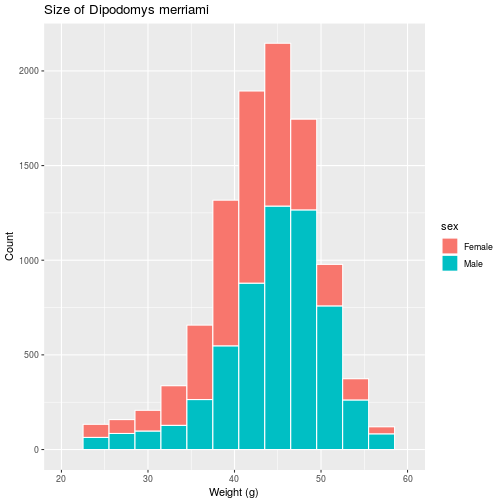If we prefer a histogram showing probability, rather than counts, as the scale on the vertical axis, the aesthetic itself must be modified to include this non-default mapping for the y element.

histo <- ggplot(animals_dm,
aes(x = weight,
y = stat(density),
fill = sex)) +
geom_histogram(binwidth = 3,
color = 'white') +
labs(title =
'Size of Dipodomys merriami',
x = 'Weight (g)',
y = 'Density')

> histo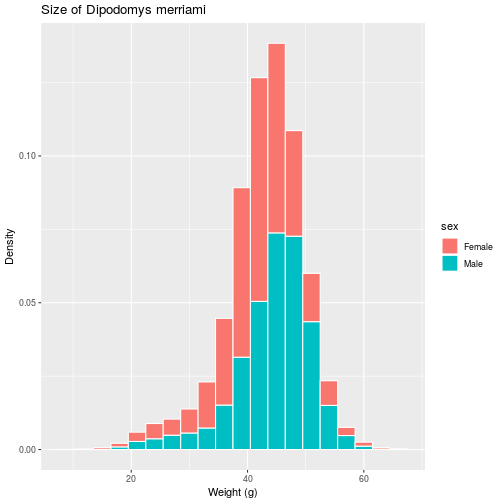Many plot-level options in ggplot2, from background color to font sizes, are defined as part of “themes”. The next modification to histo changes the base theme of the plot to theme_bw (replacing the default theme_grey) and sets a few options manually with the theme function.

histo <- histo + theme_bw() + theme(
legend.position = c(0.2, 0.5),
plot.title = element_text(
face = 'bold', hjust = 0.5),
axis.title.y = element_text(
size = 13, hjust = 0.1),
axis.title.x = element_text(
size = 13, hjust = 0.1))

> histo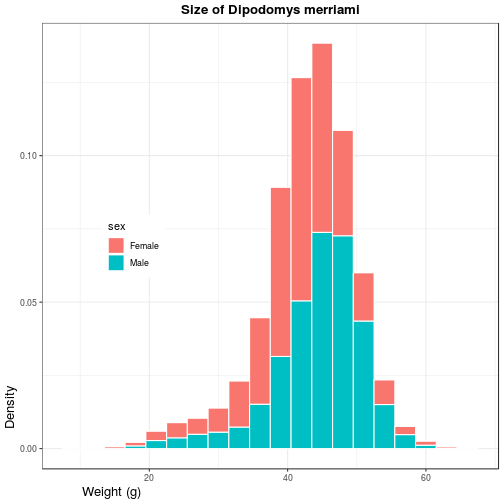Use ?theme for a list of available theme options. Note that position (both legend.position and hjust for horizontal justification) should be given as a proportion of the plot window (i.e. between 0 and 1).

Top of Section

## Facets

To conclude this overview of ggplot2, we’ll apply the same plotting instructions to different subsets of the data, creating panels or “facets”. The facet_wrap function takes a vars argument that, like the aes function relates a variable in the dataset to a visual element, the panels.

animals_common <- filter(animals,
species_id %in% c('DM', 'PP', 'DO'))
faceted <- ggplot(
animals_common, aes(x = weight)) +
geom_histogram() +
facet_wrap(vars(species_id)) +
labs(title =
'Weight of most common species',
x = 'Count',
y = 'Weight (g)')

> faceted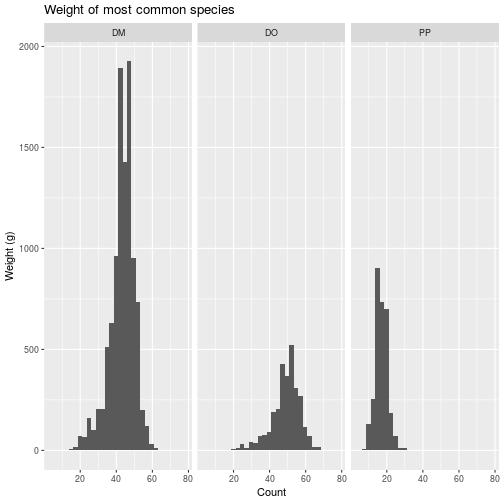The un-grouped data may be added as a layer on each panel, but you have to drop the grouping variable (i.e. month).

faceted_all <- faceted +
geom_histogram(data =
select(animals_common, -species_id),
alpha = 0.2)

> faceted_all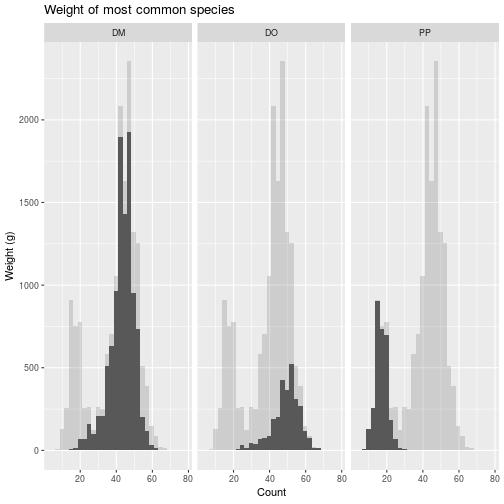Top of Section

## Review

1. Call ggplot with parameters data and aes paving the way for subsequent layers.
2. Add one or more geom_* layers, possibly with data transformations.
3. Add labs to annotate your plot and axes labels.
4. Optionally, add scale_*, theme_*, facet_*, or other modifiers that work on underlying layers.

Top of Section

## Exercises

### Exercise 1

Use dplyr to filter down to the animals with species_id equal to DM. Use ggplot to show how the mean weight of this species changes each year, showing males and females in different colors. (Hint: Baby steps! Start with a scatterplot of weight by year. Then expand your code to plot only the means. Then try to distinguish sexes.)

View solution

### Exercise 2

Create a histogram, using a geom_histogram layer, of the weights of individuals of species DM and divide the data by sex. Note that instead of using color in the aesthetic, you’ll use fill to distinguish the sexes. To silence that warning, open the help with ?geom_histogram and determine how to explicitly set the bin width.

View solution

### Exercise 3

The facet_grid layer (different from facet_wrap) requires an argument for both row and column varaibles, creating a grid of panels. For these three common animals, create facets in the weight histogram along two categorical variables, with a row for each sex and a column for each species.

View solution

## Solutions

### Solution 1

animals_dm <- filter(animals, species_id == 'DM')
ggplot(animals_dm,
aes(x = year, y = weight, color = sex)) +
geom_line(stat = 'summary',
fun.y = 'mean')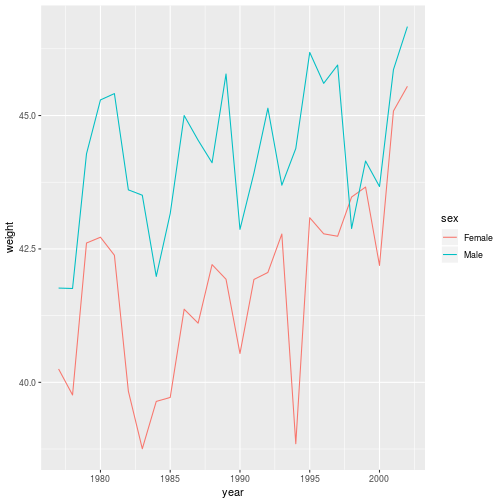Return

### Solution 2

filter(animals, species_id == 'DM') %>%
ggplot(aes(x = weight, fill = sex)) +
geom_histogram(binwidth = 1)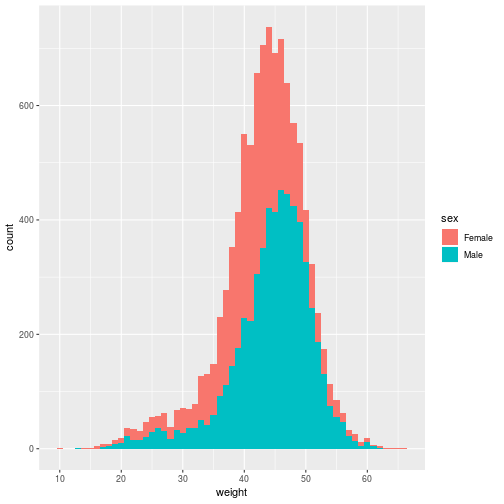Return

### Solution 3

ggplot(animals_common,
aes(x = weight)) +
geom_histogram() +
facet_grid(vars(sex), vars(species_id)) +
labs(title = 'Weight of common species by sex',
x = 'Count',
y = 'Weight (g)')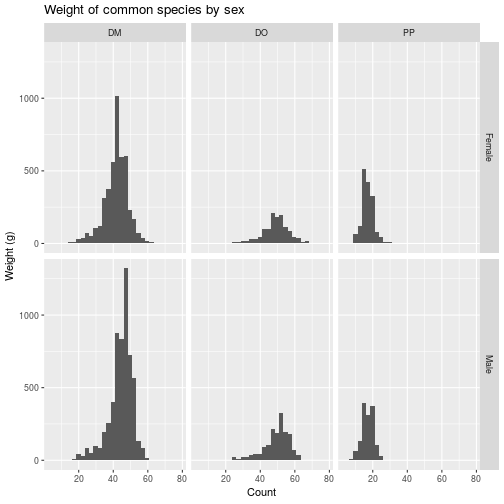Return

Top of Section

If you need to catch-up before a section of code will work, just squish it's 🍅 to copy code above it into your clipboard. Then paste into your interpreter's console, run, and you'll be ready to start in on that section. Code copied by both 🍅 and 📋 will also appear below, where you can edit first, and then copy, paste, and run again.

# Nothing here yet!